Updated by arclark on Mar 10, 2017
REPORT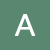arclark
Owner
10 items   1 followers   0 votes   3 views

# Parallel and Perpendicular Lines

This list contains OERs on parallel and perpendicular lines and parallel lines cut by a transversal.

1

## Parallel and perpendicular lines (OpenAlgebra.com)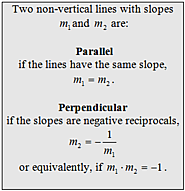This OER is from Open Algebra. It has good graphics and videos explaining slope and if two lines are parallel and perpendicular. It shows the work when writing in slope-intercept form and gives steps.

2

## Finding Equations of Parallel and Perpendicular Lines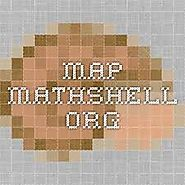This website is for students to find the relationship between parallel and perpendicular lines. The website gives an introduction, materials needed, time needed and resources. The lesson encourages discussions.

3

## Parallel & perpendicular lines from graph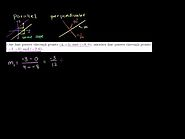This video explains if lines are parallel or perpendicular by finding the slopes of lines passing through certain points. There is practice for the student after the video.

4

## Vocabulary Parallel, Perpendicular, and Skew Lines and Parallel Planes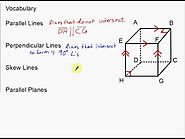This video looks a 3-D object instead of a coordinate plane and defines vocabulary words.

5

## 8.3c Parallel and Perpendicular Lines - Perpendicular Equations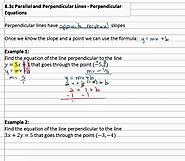I like how this video explains how to find the equation of a line that is perpendicular to a certain line and goes through a certain point.

6

## Writing the Point Slope Form of the Equation of a Line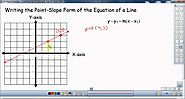This video explains how to write an equation of a line in Point-Slope Form instead of slope-intercept form. It has good graphics.

8

## OER Fellowship Project: City planning with parallel lines"This project introduces students to parallel lines and angles through videos, practice exercises, simulations, hands-on activities, and student presentations." The simulations were great. This will be a good project for students.

9

## Parallel Lines: Find the Missing Angle"You'll practice finding the missing angles using the properties of parallel lines." This is a fun activity for students. It will keep them engaged.

11

## MyOpenMath Assessment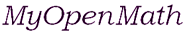"Are you a student looking to study mathematics on your own, and want to do exercises with immediate feedback as you work through a free and open textbook? Then read more about our self study courses." This is an interactive website. It is fun to play.

12

## Angles, parallel lines, & transversals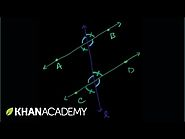In this video you will learn about parallel lines, transversals, and the angles they form.